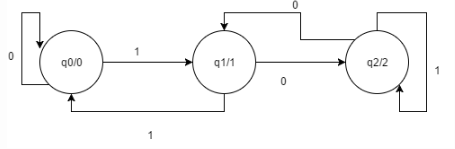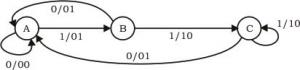Courses

# Test: Moore Machine

## 10 Questions MCQ Test Theory of Computation | Test: Moore Machine

Description
This mock test of Test: Moore Machine for Computer Science Engineering (CSE) helps you for every Computer Science Engineering (CSE) entrance exam. This contains 10 Multiple Choice Questions for Computer Science Engineering (CSE) Test: Moore Machine (mcq) to study with solutions a complete question bank. The solved questions answers in this Test: Moore Machine quiz give you a good mix of easy questions and tough questions. Computer Science Engineering (CSE) students definitely take this Test: Moore Machine exercise for a better result in the exam. You can find other Test: Moore Machine extra questions, long questions & short questions for Computer Science Engineering (CSE) on EduRev as well by searching above.
QUESTION: 1

### Moore Machine is an application of:

Solution:

Finite automaton with an output is categorize din two parts: Moore M/C and Mealy M/C.

QUESTION: 2

### In Moore machine, output is produced over the change of:

Solution:

Moore machine produces an output over the change of transition states while mealy machine does it so for transitions itself.

QUESTION: 3

### For a give Moore Machine, Given Input=’101010’, thus the output would be of length:

Solution:

Initial state, from which the operations begin is also initialized with a value.

QUESTION: 4

Statement 1: Null string is accepted in Moore Machine.
Statement 2: There are more than 5-Tuples in the definition of Moore Machine.

Choose the correct option:

Solution:

Even ε, when passed as an input to Moore machine produces an output.

QUESTION: 5

The total number of states and transitions required to f orm a moore machine that will produce residue mod 3.

Solution:QUESTION: 6

The Finite state machine described by the following state diagram with A as starting state, where an arc label is x / y and x stands for 1-bit input and y stands for 2- bit outputSolution:

We assume the input string to be 1101.

1. (A, 1) –> (B, 01)
Here, previous input bit + present input bit = 0 + 1 = 01 = output

2. (B, 1) –> (C, 10)
Here, previous input bit + present input bit = 1 + 1 = 10 = output

3. (C, 0) –> (A, 01)
Here, previous input bit + present input bit = 1 + 0 = 01 = output

4. (A, 1) –> (B, 01)
Here, previous input bit + present input bit = 0 + 1 = 01 = output

Thus, option (A) is correct.

QUESTION: 7

What is the output for the given language?
Language: A set of strings over ∑= {a, b} is taken as input and it prints 1 as an output “for every occurrence of a, b as its substring. (INPUT: abaaab)

Solution:

The outputs are as per the input, produced.

QUESTION: 8

The output alphabet can be represented as:

Solution:

Source-The tuple definition of Moore and mealy machine comprises one new member i.e. output alphabet as these are finite machines with output.

QUESTION: 9

The O/P of Moore machine can be represented in the following format:

Solution:

Op(t)=δ(Op(t)) is the defined definition of how the output is received on giving a specific input to Moore machine.

*Multiple options can be correct
QUESTION: 10

Which of the following is a correct statement?

Solution: